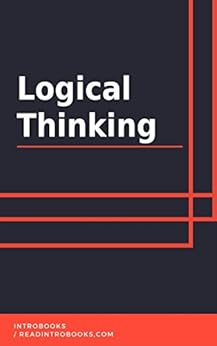Logic is an important part of the lives of humans and it is something which has helped the human race so far, for understanding various concepts of nature and their man-made things and inventions. Logic is a technique which can be applied to any stream, where humans work. Logic is used for a better understanding of a concept. The concept can be a simple problem or a complex one. This can be varying from problems to problems. Here, a precise discussion is present to understand the major concept behind the logic. Logic is not merely a word but it is a proficient technique for solving the problem. Here, the various arguments of the logic are also discussed in order to understand the application of the right kind of logic to a particular problem. Apart from this, there will also be a section of the different types of logics which are most common t humans and are used most commonly used. The data provided, will be beneficial in order to understand the various reasoning and arguments of logic and the significance of logic in the daily life of a human as well as its importance in the professional life.

Ebook \$2.99 Print \$7.99 Audiobook \$3.99

Tagged: